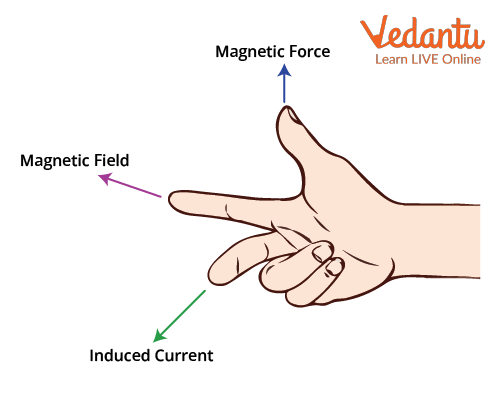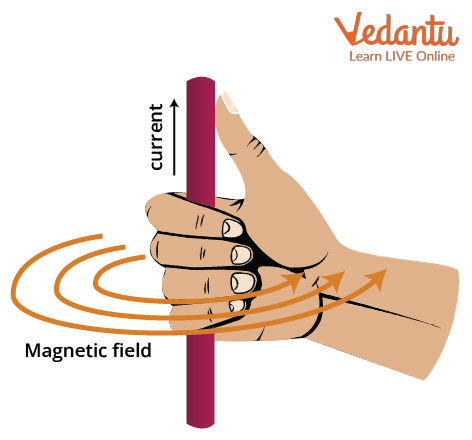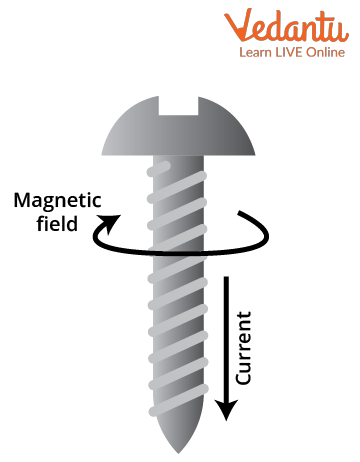Courses
Courses for Kids
Free study material
Free LIVE classes
More

# Right-Hand Thumb Rule | JEE Important TopicLIVE
Join Vedantu’s FREE Mastercalss

## Introduction to Fleming’s Right Hand Thumb Rule

Fleming’s right-hand thumb rule was discovered and developed in the late 19th century by Mr John Fleming to explore the direction of induced current in magnetism and electromagnetism. In essence, in Math or Physics, this rule is commonly used as a mnemonic to comprehend the orientation of the three axes, X, Y and Z in a three-dimensional plane. Two prominent people are well-known for discovering and further developing this concept.

One is Mr Fleming for discovering Fleming’s right-hand thumb rule and the other is Mr Maxwell for developing Maxwell’s right-hand thumb rule. These rules explain the concept which is needed to find the direction of magnetic lines of flux, the direction of the magnetic force acting on the moving charges and also the direction of the current produced by electromagnetic activity.

Let us discuss every rule, how they work and their applications in the electromagnetic fields.

## Fleming’s Right Hand Thumb Rule - Diagram

Fleming’s Right Hand Thumb Rule states that if the thumb, index finger and middle finger of the right hand are set such that they are perpendicular to each other, then the direction pointed by the thumb is the magnetic force that is being exerted on the charges in a magnetic system and the direction pointed by the index finger gives us the flow of magnetic flux field lines and lastly middle finger tells us about the direction of the current induced in that field. This is shown in the below right hand thumb rule diagram.Right hand thumb rule diagram

This concept can be simplified in a way that, if there is an object on which a magnetic force is being exerted, then both the current induced and the magnetic field directions will be perpendicular to that of the magnetic force. This way, if one quantity’s direction is given or defined, we would be able to get the direction of the other two.

This has a wide variety of applications in the many fields of electromagnetism. In Electric Generators, this rule is seamlessly used to figure out the direction of current in the generator windings and coils. Whenever a conductor coil is placed in an electromagnetic field, an Electromotive force (EMF) gets induced in the coil. This EMF results in the production of current in a closed path. This is one application of right hand thumb rule for magnetic fields.

## Maxwell’s Right Hand Thumb Rule

Maxwell’s Right-Hand Thumb rule is very simple and vivid to understand. If a person grabs a current-carrying wire with his right hand with his thumb open and all the other fingers closed, then the thumb points in the direction of the current and the curled fingers give us the interpretation of the magnetic flux lines.

Here, magnetic flux field lines are drawn circularly in the way the curled fingers look. Let us dive more into this explanation.

If the current in a current-carrying wire is flowing upwards, then after holding the wire using maxwell’s right-hand thumb rule, you can conclude that the magnetic field flux lines are anti-clockwise. If we hold the wire upside down such that the current is flowing from top to down, then we point the thumb finger downwards which results in the clockwise direction of the magnetic field flux lines.Maxwell’s Right Hand Thumb Rule

The use of the right-hand thumb rule is that we can easily determine the relationship between the directions of the current and the magnetic field.  Importantly, this rule is also stated as the Maxwell Corkscrew rule which is almost the same. Let’s discuss this in detail.

## Maxwell Corkscrew Rule

Firstly, the corkscrew is a term used to define an object which is used to pull out a cork from a bottle. It looks like a normal screw which has a spiral and sharp end.

To understand this rule, let us assume a situation where we are pulling out a screw from the cork. If we are pulling it outside, then absolutely it rotates anti-clockwise.Maxwell’s Corkscrew rule

Hereby, inferring that if the screw is rotating anti-clockwise, it is coming out so, the current direction is towards the outside.

Similarly, if the corkscrew is rotating clockwise, the current direction is inwards. This is the same logic used in the aforementioned Maxwell's right-hand thumb rule and is considered the one application of right hand thumb rule for the magnetic field in physics.

## Conclusion

Therefore, the use of the right-hand thumb rule is very vital in electromagnetism. Fleming’s and Maxwell's rules have numerous applications. Application of the right-hand thumb rule is also instilled in electric motors as well as generators. Maxwell’s corkscrew law is very similar to Maxwell's right-hand thumb rule as they follow the same logic. Learning these rules and how they work is very essential.

Last updated date: 26th Sep 2023
Total views: 2.1k
Views today: 0.02k

## FAQs on Right-Hand Thumb Rule | JEE Important Topic

1. What is Ampere’s right-hand thumb rule in Physics?

Maxwell’s right-hand thumb rule is also called Ampere’s right-hand thumb rule as it is used majorly to determine the direction of the current. This states that if the curled fingers are in the direction of magnetic field circular flux lines, then the thumb gives us the direction of the induced current flow. It can be vice versa too. If the direction of the thumb tells the direction of the current, then the direction in which fingers get curled gives us the direction of the magnetic field.

2. What is Fleming’s left-hand thumb rule?

This rule is the same as Fleming’s right-hand rule but here we use the left hand as the reference. This also states that if a current-carrying coil is placed in an external magnetic field, then the magnetic force acting upon the coil is perpendicular to both the current and the magnetic field lines. This has the application in the working of electric motors to find the direction of motion, i.e., the direction of the magnetic force acting on the coil.